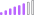cancel
Showing results for
Did you mean:Post Patron

## Weekly target not adding up to be the same as my Monthly target

hi guys,

ran into a problem with my weekly target as it is not adding up to my monthly one.

I had to tinker my monthly report so that it would fit into a weekly format.

I did that but the amounts are adding up correctly.

here is what I have done:

```Weekly Date =
var CurrentDate=LASTDATE('Calendar Table'[Date])
var DayNumberOfWeek=WEEKDAY(LASTDATE('Calendar Table'[Date]),3)
return
CurrentDate,
-1*DayNumberOfWeek,
DAY)```

This takes the first date of the week and pulls it across the week so that any dates in the date column get picked up as a week.

Targets:

```Daily Target = '20-21 Revenue Targets'[Target] / MAX('Calendar Table'[Day in Month])

20-21 Weekly Revenue Target = CALCULATE(sum('20-21 Revenue Targets'[Daily Target]), DATESMTD('Closed Calendar Table'[Date]))

20-21 Revenue Weekly Targets = [20-21 Weekly Revenue Target] * [Working days in month]```

the working days in month measure is a CALC(SUM of the working days column in my calendar table that shows 1 for workings day and 0 for non-working days.

The reason why I am not getting the same amount is that I am dividing a larger amount than I am multiplying. (100 / 30) * 20 = 66.6

what could I change in the formulas that would give me the same as my monthly target? or is there an easier way to do this?

 Desired Output Sample Weekly Date Target Weekly Target Weekly Date Target Weekly Target 01/07/2020 10,000 2000 01/07/2020 10,000 966 06/07/2020 2000 06/07/2020 1610 13/07/2020 2000 13/07/2020 1610 20/07/2020 2000 20/07/2020 1610 27/07/2020 2000 27/07/2020 1610 10,000 7406

Weekly Target = (Target / Days in Month) * Working days in Month
Weekly Target = (10,000 / 31) * 3/5
01/07/2020 weekly has 3 days in the week
The rest have 5 days

1 ACCEPTED SOLUTIONSuper User

@paulfink - This took some work, PBIX file is attached. I believe the core issue is that you were using 5 days weeks but then using a per day of all 31 days in the month. Anyway, this is the calculation (below). Can probably be simplified, I was troubleshooting as I went.

``````Measure 2 =
VAR __Date = MAX('Table'[Date])
VAR __Month = MONTH(MAX([Date]))
VAR __Year = YEAR(MAX([Date]))
VAR __Table = SELECTCOLUMNS(FILTER(ALL('Table'[Date]),MONTH([Date]) = __Month && YEAR([Date]) = __Year),"Date",[Date])
VAR __Table1 = ADDCOLUMNS(__Table1a,"Days",COUNTX(FILTER(ALL('Calendar'),WEEKDAY([Date],2) < 6 && MONTH([Date]) = [Month] && YEAR([Date]) = [Year] && WEEKNUM([Date]) = [Weeknum]),[Date]))
VAR __Target = MAXX(FILTER('Targets',[Month] = __Month),[Target])
VAR __DaysInMonth = COUNTX(FILTER(CALENDAR(DATE(__Year,__Month,1),(EOMONTH(DATE(__Year,__Month,1),0))),WEEKDAY([Date],2)<6),[Date])
VAR __PerDay = __Target / __DaysInMonth
VAR __Short = SUMX(__Table2,[Short])
VAR __WeeksShort = COUNTX(FILTER(__Table2,[Days]<5),[Date])
VAR __Weeks = COUNTX(__Table2,[Date])
VAR __FullWeeks = __Weeks - __WeeksShort
VAR __AddPerWeek = SUMX(__Table2,[Short]) / __Weeks * __PerDay
VAR __Table3 = ADDCOLUMNS(__Table2,"Target",[Days] * __PerDay )
RETURN
IF(HASONEVALUE('Table'[Date]),MAXX(FILTER(__Table3,[Date] = __Date),[Target]),SUMX(__Table3,[Target]))``````

Become an expert!: Enterprise DNA
External Tools: MSHGQM
Latest book!:
Mastering Power BI 2nd EditionDAX is easy, CALCULATE makes DAX hard...
11 REPLIES 11Super User

This looks like a measure totals problem. Very common. See my post about it here: https://community.powerbi.com/t5/DAX-Commands-and-Tips/Dealing-with-Measure-Totals/td-p/63376

Also, this Quick Measure, Measure Totals, The Final Word should get you what you need:
https://community.powerbi.com/t5/Quick-Measures-Gallery/Measure-Totals-The-Final-Word/m-p/547907

If that is not it, please first check if your issue is a common issue listed here: https://community.powerbi.com/t5/Community-Blog/Before-You-Post-Read-This/ba-p/1116882

The most important parts are:
1. Sample data as text, use the table tool in the editing bar
2. Expected output from sample data
3. Explanation in words of how to get from 1. to 2.

Become an expert!: Enterprise DNA
External Tools: MSHGQM
Latest book!:
Mastering Power BI 2nd EditionDAX is easy, CALCULATE makes DAX hard...Post Patron

@Greg_Deckler sorry this didnt helpSuper User

@paulfink - OK, can you post sample data as text and expected output?

Become an expert!: Enterprise DNA
External Tools: MSHGQM
Latest book!:
Mastering Power BI 2nd EditionDAX is easy, CALCULATE makes DAX hard...Post Patron

@Greg_Deckler it is already in my postSuper User

Refer this file

https://www.dropbox.com/s/fnq82ksdzk1lqs3/Target_allocation_daily.pbix?dl=0

Daily Target table is created from monthly

Check the date table used has working days, the same way every month add distinct weeks based on week start dates

Instead, date, take week start date and month dates  in final tableSuper User

@paulfink - This took some work, PBIX file is attached. I believe the core issue is that you were using 5 days weeks but then using a per day of all 31 days in the month. Anyway, this is the calculation (below). Can probably be simplified, I was troubleshooting as I went.

``````Measure 2 =
VAR __Date = MAX('Table'[Date])
VAR __Month = MONTH(MAX([Date]))
VAR __Year = YEAR(MAX([Date]))
VAR __Table = SELECTCOLUMNS(FILTER(ALL('Table'[Date]),MONTH([Date]) = __Month && YEAR([Date]) = __Year),"Date",[Date])
VAR __Table1 = ADDCOLUMNS(__Table1a,"Days",COUNTX(FILTER(ALL('Calendar'),WEEKDAY([Date],2) < 6 && MONTH([Date]) = [Month] && YEAR([Date]) = [Year] && WEEKNUM([Date]) = [Weeknum]),[Date]))
VAR __Target = MAXX(FILTER('Targets',[Month] = __Month),[Target])
VAR __DaysInMonth = COUNTX(FILTER(CALENDAR(DATE(__Year,__Month,1),(EOMONTH(DATE(__Year,__Month,1),0))),WEEKDAY([Date],2)<6),[Date])
VAR __PerDay = __Target / __DaysInMonth
VAR __Short = SUMX(__Table2,[Short])
VAR __WeeksShort = COUNTX(FILTER(__Table2,[Days]<5),[Date])
VAR __Weeks = COUNTX(__Table2,[Date])
VAR __FullWeeks = __Weeks - __WeeksShort
VAR __AddPerWeek = SUMX(__Table2,[Short]) / __Weeks * __PerDay
VAR __Table3 = ADDCOLUMNS(__Table2,"Target",[Days] * __PerDay )
RETURN
IF(HASONEVALUE('Table'[Date]),MAXX(FILTER(__Table3,[Date] = __Date),[Target]),SUMX(__Table3,[Target]))``````

Become an expert!: Enterprise DNA
External Tools: MSHGQM
Latest book!:
Mastering Power BI 2nd EditionDAX is easy, CALCULATE makes DAX hard...Post Patron

@Greg_Deckler this did the job!! thank you so much.

I got another challenge if you're up for it, here is the link:

https://community.powerbi.com/t5/Desktop/Calculate-the-change-in-progress-from-the-start-of-last-wee...Super User

@paulfink - If you want people to help, why would you make them re-type all of that data? Doesn't seem very nice.

Become an expert!: Enterprise DNA
External Tools: MSHGQM
Latest book!:
Mastering Power BI 2nd EditionDAX is easy, CALCULATE makes DAX hard...Post Patron

@Greg_Deckler that better?

Also, i need a column that is like the DAY function (shows 1-31 depending on date) but have it so that it doesn't count days that are not working days e.g.

it goes: 1,2,3,4,5,0,0,6,7,8,9,10,00

not: 1,2,3,4,5,6,7,8,9,10

so i can do a MAX formulaSuper User

@paulfink ,Can you share sample data and sample output in table format?Post Patron

@amitchandak i have updated my post with desired output and sample data.

Any more questions let me know